# I.E. Irodov Solutions on Electric Current

Electric Current is an important chapter in Physics that mainly deals with the electric field and properties with capacitors. The chapter comprises various numerical based on the syllabus and also the practical applications. I.E. Irodov Physics provides solutions to different varieties of numerical. Electric Current is a necessary resource element that is needed by all students to score good marks in JEE Main and entrance examinations. It is one of the best ways to study and practice for physics examinations to score high. The problems asked here are mainly based on current in a circuit, voltage, movement of charge and so on.

This chapter mainly focuses on formulas and equations based on the circuit diagrams. It helps the students to analyse which law can be applied to solve the problems. The movement of electricity in the circuit is explained and how the resistors, capacitors and other parameters play a vital role in determining the solution to the given problem. The problems and questions in this chapter mainly comprises capacitors connected in series, parallel, change in energy when electric field is applied or removed. Students can access I.E. Irodov textbook Chapter 3.4 - electric current solutions here.

### I.E. Irodov Solutions on Electric Current

1. A long cylinder with uniformly charged surface and cross-sectional radius a = 1.0 cm moves with a constant velocity v = 10 m/s along its axis. An electric field strength at the surface of the cylinder is equal to E = 0.9 kV/cm. Find the resulting convection current, that is, the current caused by mechanical transfer of a charge.

Solution:

1. The convention current is given by

$I=\frac{dq}{dt}$ --------(1)

Here, dq = λ dx, where λ is the linear charge density.

But, from the Gauss’ theorem, electric field at the surface of the cylinder,

$E=\frac{\lambda }{2\pi \varepsilon _{0}a}$

Hence, substituting the value of λ and subsequently of dq in Eqs. (1), we get

$I=\frac{2\pi \varepsilon _{0}a dx }{dt}$

$2\pi \varepsilon _{0}E av$ as $\frac{dx}{dt}=v$

2. An air cylindrical capacitor with a dc voltage V = 200 V applied across it is being submerged vertically into a vessel filled with water at a velocity v = 5.0 mm/s. The electrodes of the capacitor are separated by a distance d = 2.0 mm, the mean curvature radius of the electrodes is equal to r = 50 mm. Find the current flowing in this case along lead wires, if d ≪ r.

Solution:

1. Since d << r, the capacitance of the given capacitor can be calculated using the formula for a parallel plate capacitor. Therefore if the water (permittivity ε) is introduced up to a height x and the capacitor is of length l, we have,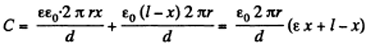Hence charge on the plate at that instant, q = CV

Again we know that the electric current intensity,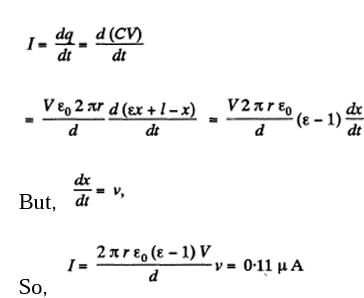3. At the temperature 0°C, the electric resistance of conductor 2 is η times that of conductor 1. Their temperature coefficients of resistance are equal to α2 and α1 respectively. Find the temperature coefficient of resistance of a circuit segment consisting of these two conductors when they are connected

1. (a) in series

2. (b) in parallel

Solution:

1. We have, Rt = R0 (1 + αf),(1)

Where Rt and R0 are resistances at t°C and 0°C respectively and a is the mean temperature coefficient of resistance.

So,

R1= Ro(1+α1t) and R2= ηRo(1+α2t)

So R = R1+R2= Ro=[(1+η)+(α1+ηα2)t]---------------------(1)

=Ro(1+η)

$Ro=[(1+\eta )\left [ 1+2(\frac{\alpha_{1} +\eta \alpha _{2}}{1+\eta })t \right ]$-------------(2)

Comparing equation (1) and (2) we conclude that temperature co-efficient of resistance of the circuit,

$\alpha =\frac{\alpha_{1} +\eta \alpha _{2}}{1+\eta }$

(b) In parallel combination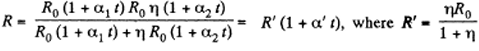Now, neglecting the terms, proportional to the product of temperature coefficients, as being very small, we get

$\alpha' =\frac{\eta \alpha_{1} +\alpha _{2}}{1+\eta }$

4. Find the resistance of a wireframe shaped as a cube (Figure given) when measured between points (a) 1-7 (b) 1-2; (c) 1-3.

The resistance of each edge of the frame is R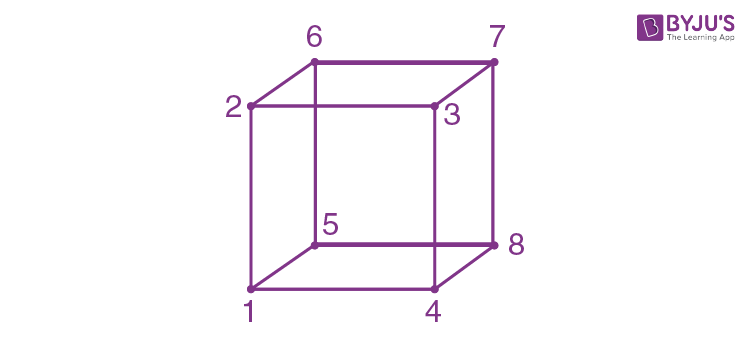Solution:

1. The currents are as shown. From Ohm's law applied between 1 and 7 via 1487 say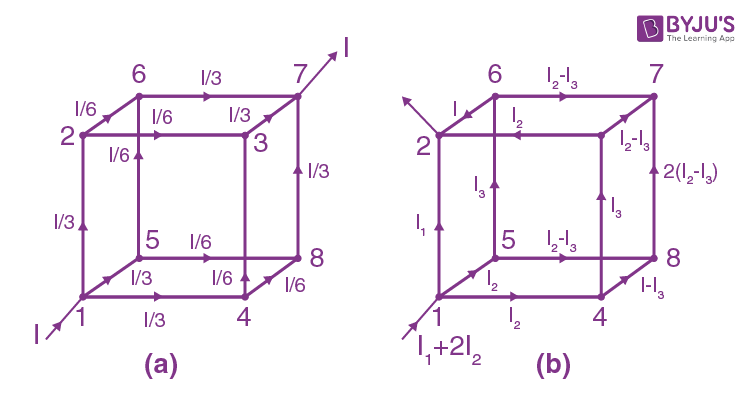IReq=$\frac{1}{3}R + \frac{1}{6}R +\frac{1}{3}R = \frac{5}{6}RI$

Thus

Req,=$\frac{5R}{6}$

(b) Between 1 and 2 from the loop 14321

I1R=2I2R+I3R

Or

I1=I3+2I2

From the loop 48734,

(I2-I3) R+ 2(I2-I3)R + (I2-I3) R=I3R

= 4(I2-I3) =I3

$I_{3}=\frac{4}{5}I_{2}$

Then

(I1+2I2)Req=$\frac{24}{5}I_{2}R_{eq}=I_{1}R=\frac{14}{5}I_{2}R$

$R_{eq}=\frac{7}{12}R$

(c)Between 1 and 3 from the loop 15621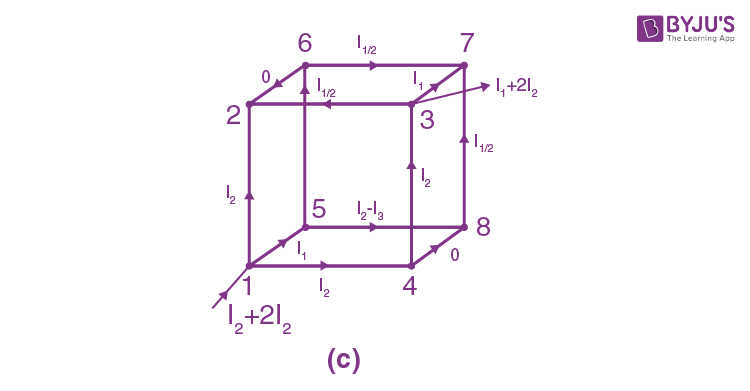$I_{2}R=I_{1}R+\frac{I_{1}}{2}R$ or $I_{2}=3\frac{I_{1}}{2}$

Then

$\left ( I_{1}+2I_{2} \right )R_{eq}=4I_{1}R_{eq}$

=$I_{2}R+I_{2}R+=3I_{1}R$

=$R_{eq}=\frac{3}{4}R$

5. At what value of the resistance Rx in the circuit shown in Figure will the total resistance between points A and B be independent of the number of cells?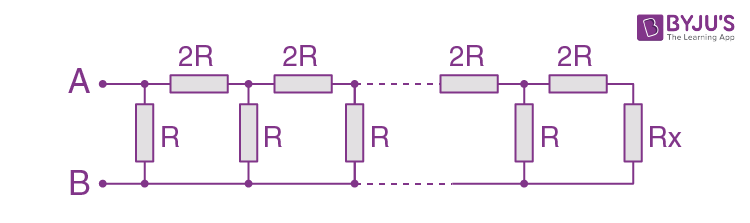Solution:

1. Total resistance of the circuit will be independent of the number of cells,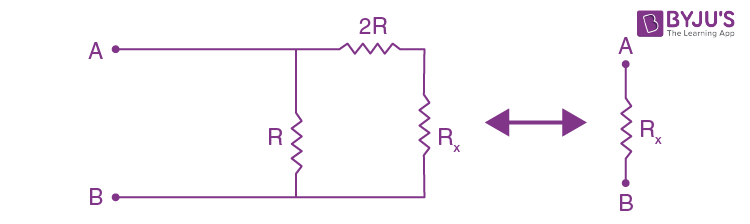If

$R_{x}=\frac{(R_{x}+2R)R}{R_{x}+2R+R}$

$R_{x}^{2}+2RR_{x}-2R^{2}=0$

On solving and rejecting the negative root of the quadratic equation, we have,

$R_{x}=R(\sqrt{3}-1)$

6. The figure shows an infinite circuit formed by the repetition of the same link, consisting of resistance R1=4.0 Ω and R2=3.0 Ω. Find the resistance of this circuit between points A and B.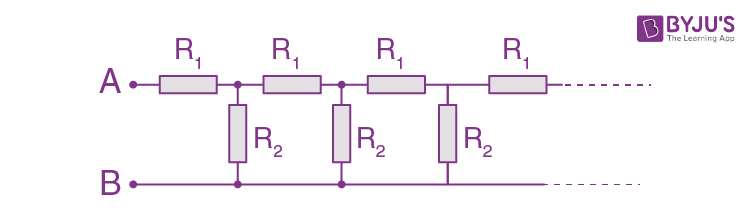Solution:

1. Let R0 be the resistance of the network,

Then

$R_{o}=\frac{R_{o} R_{2}}{R_{o}+ R_{2}}$

$R_{o}^{2}-R_{o}R_{1}-R_{1}R_{2}=0$

On solving we get

$R_{o}=\frac{R_{1}}{2}(1+\sqrt{1+4\frac{R_{2}}{R_{1}}})=6\Omega$

7. There is an infinite wire grid with square cells (Figure is given). The resistance of each wire between neighbouring joint connections is equal to R0. Find the resistance R of the whole grid between points A and B.Instruction: Make use of principles of symmetry and superposition.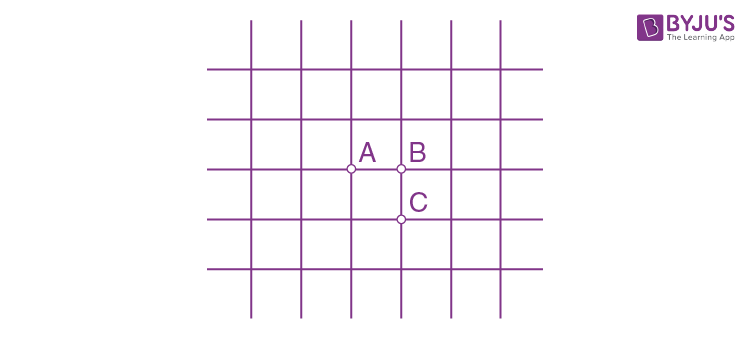Solution:

1. Suppose that the voltage V is applied between the points A and B then

V=IR=IoRo

Where R = is resistance of whole the grid,

l= the current through the grid and I, the current through the segment AB. Now from symmetry, I/4 is the part of the current, flowing through all the four-wire segments, meeting at point A and similarly, the amount of current flowing through the wires, meeting at B is also I/4. Thus a current I/2 flows through the conductor AS, i.e.

$I_{0}=\frac{I}{2}$

Hence, $R=\frac{R_{o}}{2}$

8. A homogeneous poorly conducting medium of resistivity p fills up the space between two thin coaxial ideally conducting cylinders. The radii of the cylinders are equal to a and b, with a < b, the length of each cylinder is l.

Solution:

1. Let us mentally isolate a thin cylindrical layer of inner and outer radii r and r + dr respectively. As lines of current at all the points of this layer are perpendicular to it, such a layer can be treated as a cylindrical conductor of thickness dr and cross-sectional area 2πrl. So, we have,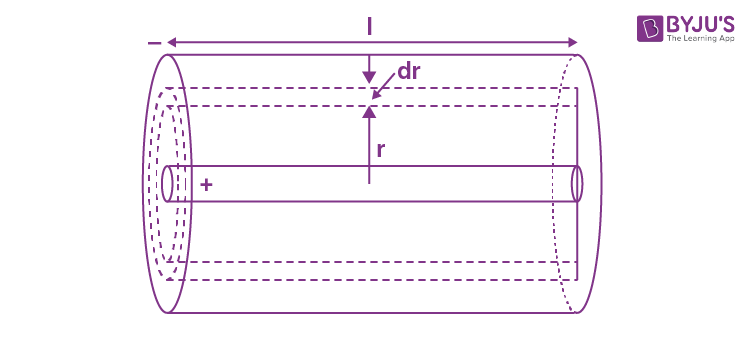$dR=\rho \frac{dr}{S(r)}=\rho \frac{dr}{2\pi rl}$

On integrating the equatio between the limits, we get

$R=\frac{p}{2\pi l}\ln \frac{b}{a}$

9. A metal ball of radius a is surrounded by a thin concentric metal shell of radius b. The space between these electrodes is filled up with a poorly conducting homogeneous medium of resistivity p. Find the resistance of the interelectrode gap. Analyse the obtained solution at $b\rightarrow \infty$

Solution:

1. Let us mentally isolate a thin spherical layer of inner and outer radii r and r + dr. Lines of current at all the points of this layer are perpendicular to it and therefore such a layer can be treated as a spherical conductor of thickness dr and cross-sectional area 4πr2. So

$dR=\rho \frac{dr}{4\pi r^{2}}$-------------(i)

On integrating (i) between the limits and b we get

$R=\frac{\rho }{4\pi}\left [ \frac{1}{a} -\frac{1}{b}\right ]$

Now for →∞

$R=\frac{\rho }{4\pi a}$

10.The space between two conducting concentric spheres of radii a and b(a<b) is filled up with homogeneous poorly conducting medium. The capacitance of such a system equals C. Find the resistivity of the medium if the potential difference between the spheres, when they are disconnected from an external voltage, decreases it-fold during the time interval Δt.

Solution:

1. In our system, resistance of the medium

$R=\frac{\rho }{4\pi}\left [ \frac{1}{a} -\frac{1}{b}\right ]$

where p is the resistivity of the medium

The current

$I=\frac{\phi }{R}=\frac{\phi }{\frac{\rho }{4\pi}\left [ \frac{1}{a} -\frac{1}{b}\right ]}$

Also

$i=\frac{dq}{dt}=\frac{-d(c\phi )}{dt}=-C\frac{d\phi }{dt}$

as capacitance is constant

On equating both the equations, we get

$\frac{\phi }{\frac{\rho }{4\pi}\left [ \frac{1}{a} -\frac{1}{b}\right ]}=-C\frac{d\phi }{dt}$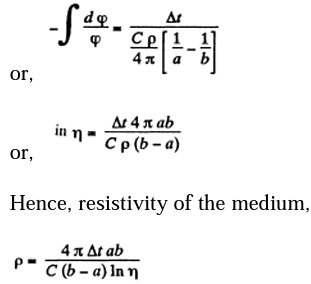11. Two metal balls of the same radius a, are located in a homogeneous poorly conducting medium with resistivity p. Find the resistance of the medium between the balls provided that the separation between them is much greater than the radius of the ball.

Solution:

1. Let us mentally impart the charge +q and - q to the balls respectively. The electric field strength at the surface of a ball will be determined only by its own charge and the charge can be considered to be uniformly distributed over the surface because the other ball is at an infinite distance. The magnitude of the field strength is given by

$E=\frac{q}{4\pi \varepsilon _{o}a^{2}}$

So current density

$j=\frac{1}{\rho }\frac{q}{4\pi \varepsilon _{o}a^{2}}$

$I=\int \vec{j}\vec{dS}=jS=\frac{q}{4\pi \varepsilon _{o}a^{2}}4\pi a^{2}=\frac{q}{\rho \varepsilon _{0}}$

But, the potential difference between the balls

$\phi_{+}-\phi _{-}=2\frac{q}{4\pi \varepsilon _{o}a}$

Hence, the sought resistance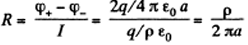12. A metal ball of radius a is located at a distance l from an infinite ideally conducting plane. The space around the ball is filled with a homogeneous poorly conducting medium with resistivity p. In the case of a≪ l find :

1. (a) the current density at the conducting plane as a function of distance r from the ball if the potential difference between the ball and the plane is equal to V

2. (b) the electric resistance of the medium between the ball and the plane

Solution:

1. The potential in the unshaded region beyond the conductor as the potential of the given charge and its image and has the form

$\phi=A(\frac{1}{\frac{1}{r_{1}}-\frac{1}{r_{2}}})$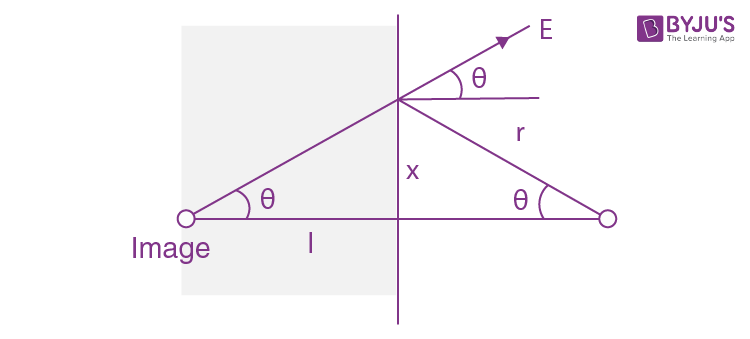Where r1 and r2 are High distances of the point from the charge and its image. The potential has been taken to be zero on the conducting plane and on the ball

$\phi\approx A(\frac{1}{a}-\frac{1}{2l})=V$

So A ≈ Va. In this calculation the conditions a << l is used to ignore the variation of φ over the ball. The electric field at P can be calculated similarly. The charge on the ball is

And

$E_{p}=\frac{V_{a}}{r^{2}}2\cos \Theta =\frac{2alv}{r^{3}}$

And also

$j=\frac{1}{\rho }E=\frac{2alV}{\rho r^{3}}$ normal to the plane.

(b) The total current flowing into the conducting plane is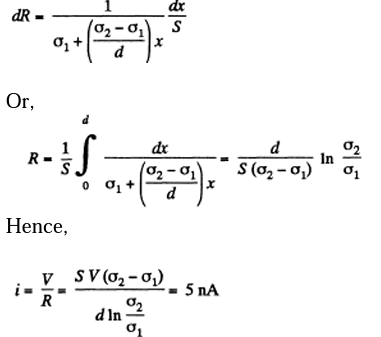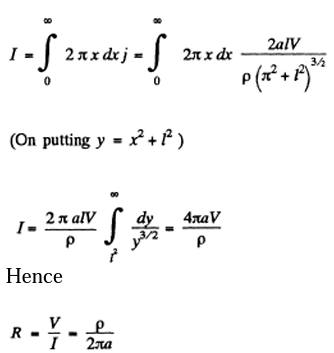13. Two long parallel wires are located in a poorly conducting medium with resistivity p. The distance between the axes of the wires is equal to l, the cross-section radius of each wire equals a. In the case a≪ l find:

1. (a) the current density at the point equally removed from the axes of the wires by a distance r if the potential difference between the wires is equal to V;

2. (b) the electric resistance of the medium per unit length of the wires.

Solution:

1. The wires themselves will be assumed to be perfect conductors so the resistance is entirely due to the medium. If the wire is of length L, the resistance R of the medium is $\alpha \frac{1}{L}$ because different sections of the wire are connected in parallel (by the medium) rather than in series. Thus if R1 is the resistance per unit length of the wire then

R = R 1 / L, Unit of R1 is ohmmeter.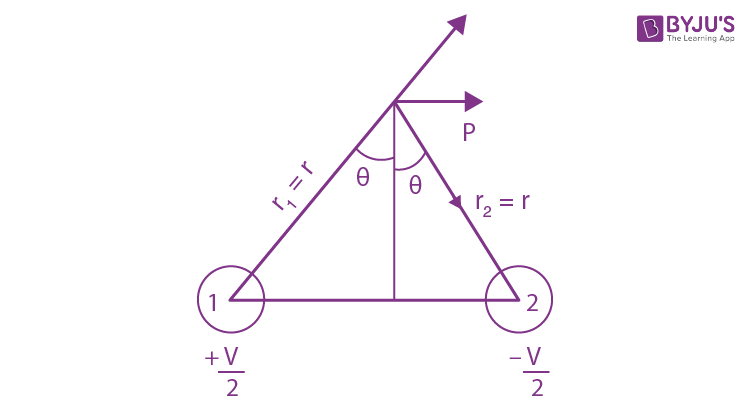The potential at a point P is by symmetry and superposition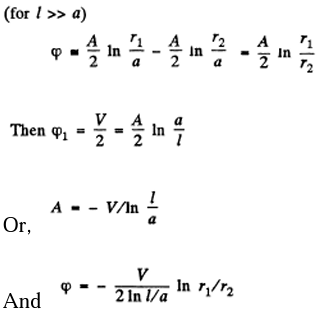We then calculate the field at a point P, which is equidistant from 1 & 2 and at a distance r from both

$E=\frac{V}{2\ln \frac{l}{a}}\frac{1}{r} 2\sin \Theta$

$=\frac{Vl}{2\ln \frac{l}{a}}\frac{1}{r^{2}}$

And

$J=\sigma E=\frac{1}{\rho }\frac{Vl}{2\ln \frac{l}{a}}\frac{1}{r^{2}}$

(b) Near either wire

$E=\frac{V}{2\ln \frac{l}{a}}\frac{1}{a}$

And

$J=\sigma E=\frac{1}{\rho}\frac{V}{2\ln \frac{l}{a}}\frac{1}{a}$

Then

$I=\frac{V}{R}=L=\frac{V}{R_{1}}=J2\pi aL$

$R_{1}=\frac{\rho }{\pi }\ln \frac{l}{a}$

14. The gap between the plates of a parallel-plate capacitor is filled with glass of resistivity p = 100 GΩ•m. The capacitance of the capacitor equals C = 4.0 nF. Find the leakage current of the capacitor when a voltage V = 2.0 kV is applied to it.

Solution:

1. Let us mentally impart the charges +q and - q to the plates of the capacitor. Then capacitance of the network,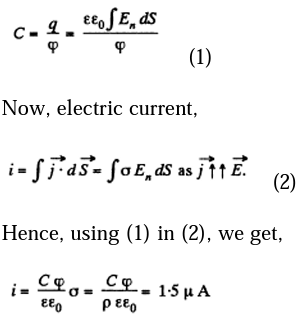15. Two conductors of arbitrary shape are embedded into an infinite homogeneous poorly conducting medium with resistivity p and permittivity e. Find the value of a product RG for this system, where R is the resistance of the medium between the conductors, and C is the mutual capacitance of the wires in the presence of the medium.

Solution:

1. Let us mentally impart charges +q and -q to the conductors. As the medium is poorly conducting, the surfaces of the conductors are equipotential and the field configuration is the same as in the absence of the medium. Let us surround, for example, the positively charged conductor, by a closed surface 5 just containing the conductor.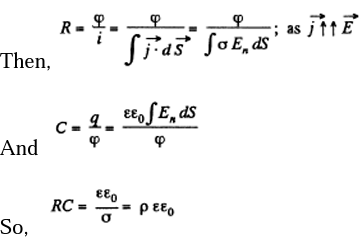16. A conductor with resistivity p bounds on a dielectric with permittivity a. At a certain point A at the conductor's surface the electric displacement equals D, the vector D being directed away from the conductor and forming an angle α with the normal of the surface. Find the surface density of charges on the conductor at point A and the current density in the conductor in the vicinity of the same point.

Solution:

1. The dielectric ends in a conductor. It is given that on one side (the dielectric side) the electric displacement D is as shown. Within the conductor, at any point A, there can be no normal component of the electric field. For if there were such a field, a current will flow towards depositing charge there which in turn will set up countering electric field causing the normal component to vanish. Then by Gauss theorem, we easily derive σ = Dn = D cos α where a is the surface charge density at A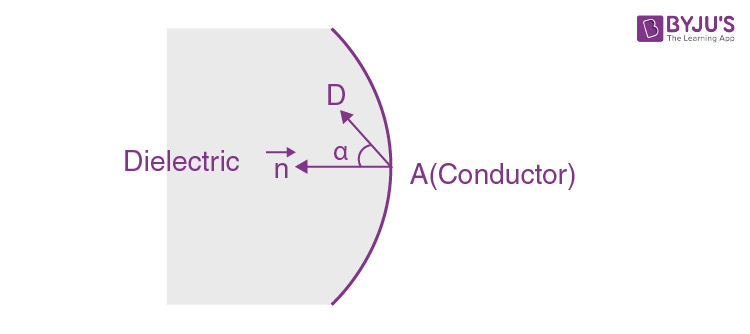The tangential component is determined from the circulation theorem

$\phi =\vec{E}.d\vec{r}=0$

It must be continuous across the surface of the conductor. Thus, inside the conductor, there is a tangential electric field of magnitude

$\frac{D \sin \alpha }{\varepsilon _{o}\varepsilon }$ at A

This implies a current, by Ohm’s law, of

$j=\frac{D \sin \alpha }{\varepsilon _{o}\varepsilon\rho }$

17. The gap between the plates of a parallel-plate capacitor is filled up with an inhomogeneous poorly conducting medium whose conductivity varies linearly in the direction perpendicular to the plates from σ1 = 1.0 pS/m to σ2 = 2.0 pS/m. Each plate has an area S = 230 cm2, and the separation between the plates is d = 2.0 mm. Find the current flowing through the capacitor due to a voltage V = 300 V.

Solution:

1. The resistance of a layer of the medium, of thickness dx and at a distance x from the first plate of the capacitor is given by,

$dR=\frac{1}{\sigma x}\frac{dx}{S}$ -------------(1)

Now, since aviaries linearly with the distance from the plate. It may be represented as

$\sigma =\sigma_{1}(\frac{\sigma _{2}-\sigma _{1}}{d})x$ at a distance x from any one of the plate.

From equation (1)18. Demonstrate that the law of refraction of direct current lines at the boundary between two conducting media has the form tan α2/tan α1 = σ21, where σ1 and σ2 are the conductivities of the media, α2 and α1 are the angles between the current lines and the normal of the boundary surface.

Solution:

1. By charge conservation, current j, leaving the medium (1) must enter the medium (2).

Thus

J 1 cosα1=J 2 cosα2

E1=E2

Which is a consequence of

$\phi \vec{E}.d\vec{r}=0$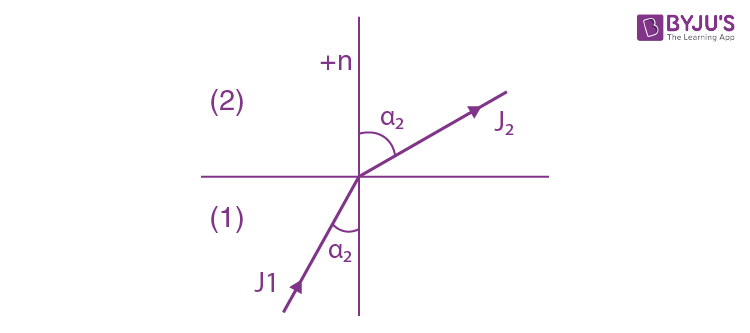Thus

$\frac{1}{\sigma _{1}}j_{1}\sin \alpha_{1}=\frac{1}{\sigma _{2}}j_{2}\sin \alpha_{2}$

$\frac{\tan \alpha _{1}}{\sigma _{1}}=\frac{\tan \alpha _{2}}{\sigma _{2}}$

$\frac{\tan \alpha _{1}}{\tan \alpha _{2}}=\frac{\sigma _{1}}{\sigma _{2}}$

19. Two cylindrical conductors with equal cross-sections and different resistivities p1 and p2 are put end to end. Find the charge at the boundary of the conductors if a current I flows from conductor 1 to conductor 2.

Solution:

1. The electric field in conductor 1 is

$E_{1}=\frac{\rho _{1}I}{\pi R^{2}}$

And that in 2 is

$E_{2}=\frac{\rho _{2}I}{\pi R^{2}}$

Applying Gauss’ theorem to a small cylindrical pill-box at the boundary.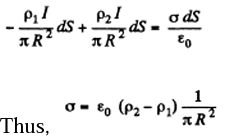And charge at the boundary=$\varepsilon _{0}(\rho _{2}-\rho _{1})I$

20. The gap between the plates of a parallel-plate capacitor is filled up with two dielectric layers 1 and 2 with thicknesses d1 and d2, permittivity’s ε1 and ε2, and resistivities p1 and p2. A de voltage V is applied to the capacitor, with an electric field directed from layer 1 to layer 2. Find σ, the surface density of extraneous charges at the boundary between the dielectric layers, and the condition under which σ = 0.

Solution:

1. We have

E1d1+E2d2=V

$\frac{1}{\rho_{1}} E_{1}=\frac{1}{\rho_{2}} E_{2}$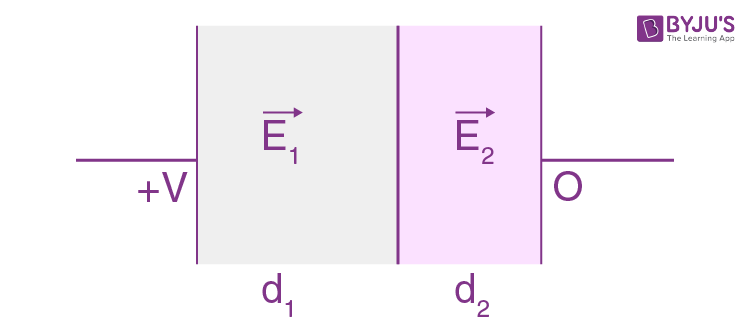Thus,

$E_{1}=\frac{\rho _{1}V}{\rho _{1}d_{1}+\rho _{2}d_{2}}$

$E_{2}=\frac{\rho _{2}V}{\rho _{1}d_{1}+\rho _{2}d_{2}}$

$\sigma= D_{2}-D_{1}=\varepsilon _{0}\varepsilon _{2}E_{2}-\varepsilon _{0}\varepsilon _{1}E_{1}$

$\frac{\varepsilon _{0}V}{\rho _{1}d_{1}+\rho _{2}d_{2}}(\varepsilon _{2}\rho _{2}-\varepsilon _{1}\rho _{1})$